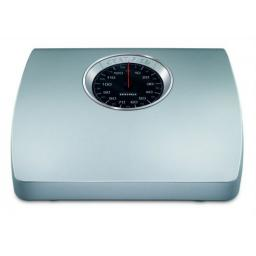# Kilograms 5994

The courier brought four packages to the company that weighed 3.5 kg, 2 1/5 kg, 3/4 kg, and 250 g. How much did all the packages weigh together? Give the result in kilograms and write it as a decimal number.

m =  6 710 = 67/10 kg

### Step-by-step explanation:Did you find an error or inaccuracy? Feel free to write us. Thank you!

Tips for related online calculators
Need help calculating sum, simplifying, or multiplying fractions? Try our fraction calculator.
Need help with mixed numbers? Try our mixed-number calculator.
Do you want to convert mass units?